Chat Now

# Two vertical aluminum rods attached at C and D support the rigid bar ABCD which has negligible weight The rods are initially stress free and the bar ABCD is initially horizontal prior to the

### Two vertical aluminum rods attached at C and D support the rigid bar ABCD which has negligible weight The rods are initially stress free and the bar ABCD is initially horizontal prior to the

Two vertical aluminum rods attached at C and D support the rigid bar ABCD which has negligible weight.

The rods are initially stress-free and the bar ABCD is initially horizontal prior to the application of the couple moment Co
Assume a value of 10 x 106 psi for the modulus of elasticity for aluminum.

Based on the information provided, select the most appropriate responses to the following: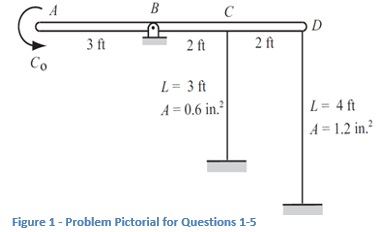1.

For an applied couple Co = 90 kip-ft the average normal strain in the vertical rod connected to point C is equal to:

A)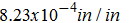B)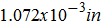C)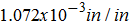D) None of the choices are within 10% of the correct result

2.

For an applied couple Co = 90 kip-ft the change in length of the vertical rod connected to point D will be:

A) None of the choices are within 10% of the correct result
B) Elongation of 0.125 inches
C) Elongation of 0.0077 inches
D) Elongation of 0.093 inches

3.

For an applied couple Co = 90 kip-ft the magnitude of the resultant reaction force at B is equal to:

A) 45000 lbf
B) 25.7 kips
C) None of the choices are within 10% of the correct result
D) 38.4 kips

4.

For an applied couple Co = 90 kip-ft and a working shear stress limit of 50 ksi the minimum required diameter of the pivot pin at B, based on a single shear configuration, will be:

A) 1.02 inches
B) 0.802 inches
C) 0.683 inches
D) None of the choices are within 10% of the correct result

5.

For an applied couple Co = 90 kip-ft the rigid bar ABCD will rotate about pin B by:

A) None of the choices are within 10% of the correct result
B) A total of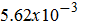radians counterclockwise
C) A total of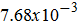degrees counterclockwise
D) A total ofdegrees counterclockwise

rahul 09-Jul-2020Get solution